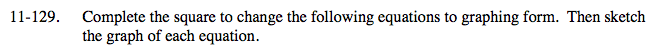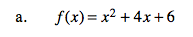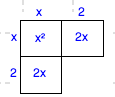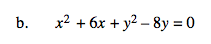### Home > A2C > Chapter 11 > Lesson 11.3.1 > Problem11-129

11-129.How many ones do you need to make a perfect square? How many do you have?

f(x) = (x + 2)2 + 2See part (a).

(x + 3)2 + (y − 4)2 = 25
Remember to sketch the equation.